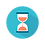From: Bayesian Models for Astrophysical Data, Cambridge Univ. Press

(c) 2017,  Joseph M. Hilbe, Rafael S. de Souza and Emille E. O. Ishida

you are kindly asked to include the complete citation if you used this material in a publication

Code 10.10 Beta model in R using JAGS, for accessing the relationship between the baryon fraction in atomic gas and galaxy stellar mass

==================================================================================

require(R2jags)

# Estimate F_gas
Fgas0\$fgas <- Fgas0\$M_HI/(Fgas0\$M_HI+Fgas0\$M_STAR)

# Prepare data to JAGS
N = nrow(Fgas0)
y <- Fgas0\$fgas
x <- log(Fgas0\$M_STAR,10)
X <- model.matrix(~ 1 + x)
K <- ncol(X)

beta_data <- list(Y = y,
X = X,
K = K,
N = N)

# Fit
Beta <-"model{
# Diffuse normal priors for predictors
for(i in 1:K){
beta[i] ~ dnorm(0, 1e-4)
}

# Diffuse prior for theta
theta~dgamma(0.01,0.01)

# Likelihood function
for (i in 1:N){
Y[i] ~ dbeta(a[i],b[i])
a[i] <- theta*pi[i]
b[i] <- theta*(1-pi[i])
logit(pi[i]) <- eta[i]

eta[i] <- inprod(beta[],X[i,])
}
}"

# Define initial values
inits <- function () {
list(beta = rnorm(2, 0, 0.1),
theta = runif(1,0,100))
}

# Identify parameters
params <- c("beta","theta")

Beta_fit <- jags(data = beta_data,
inits = inits,
parameters = params,
model = textConnection(Beta),
n.thin = 1,
n.chains = 3,
n.burnin = 5000,
n.iter = 7500)

# Output
print(Beta_fit,intervals=c(0.025, 0.975), justify = "left", digits=2)

==================================================================================Output on screen:

Inference for Bugs model at "3", fit using jags,

3 chains, each with 7500 iterations (first 5000 discarded)

n.sims = 7500 iterations saved

mu.vect       sd.vect            2.5%         97.5%       Rhat      n.eff

beta              9.30           0.15            9.04             9.58        1.07         34

beta            -0.98            0.01           -1.00           -0.95        1.07         34

theta               11.72            0.38          10.98           12.47       1.00       940

deviance   -2331.18            2.28     -2333.74      -2325.38       1.01       330

For each parameter, n.eff is a crude measure of effective sample size,

and Rhat is the potential scale reduction factor (at convergence, Rhat=1).

DIC info (using the rule, pD = var(deviance)/2)

pD = 2.6 and DIC = -2328.6

DIC is an estimate of expected predictive error (lower deviance is better).